# 二、简单数字的奇特技巧

## 标量、向量和空间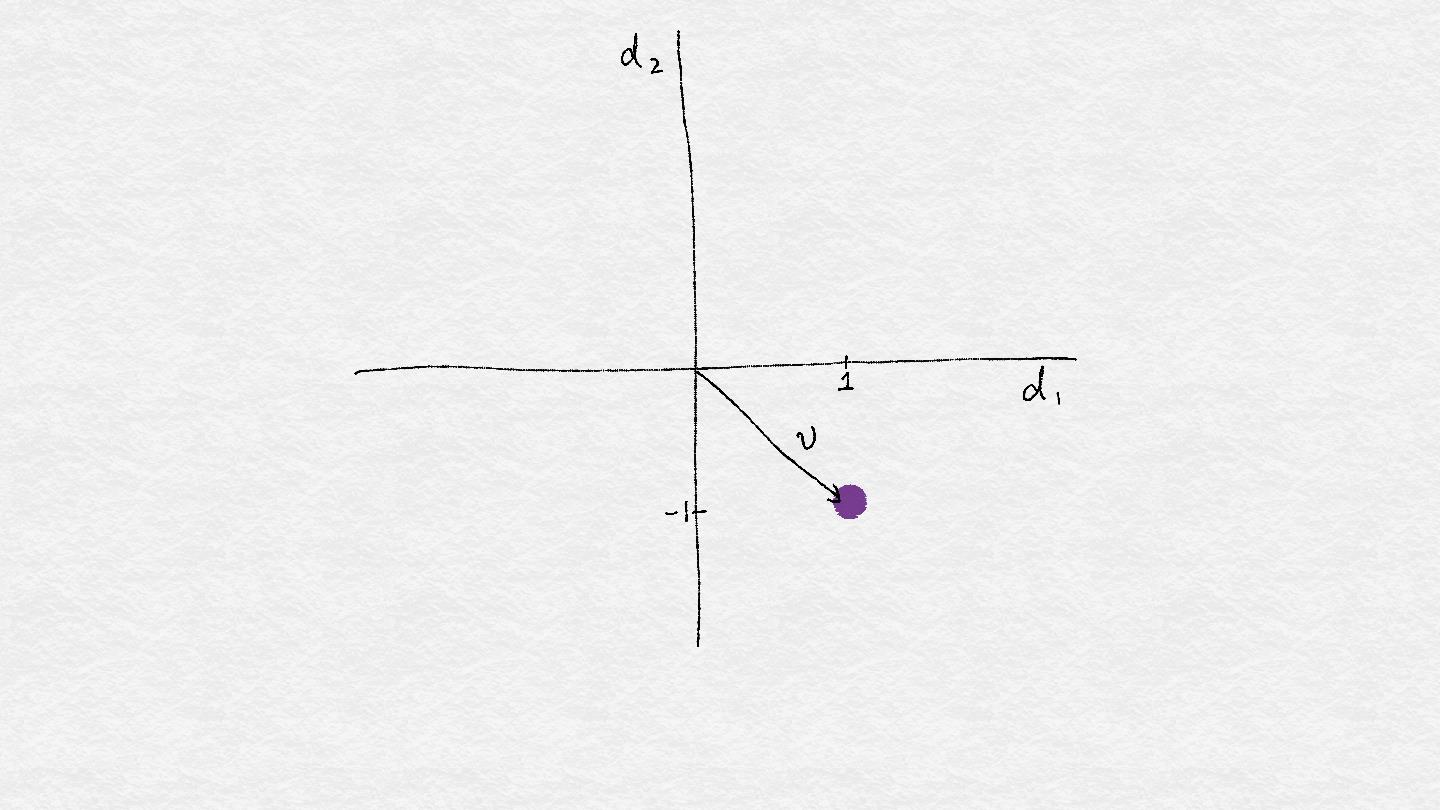Figure 2-1. A single vector.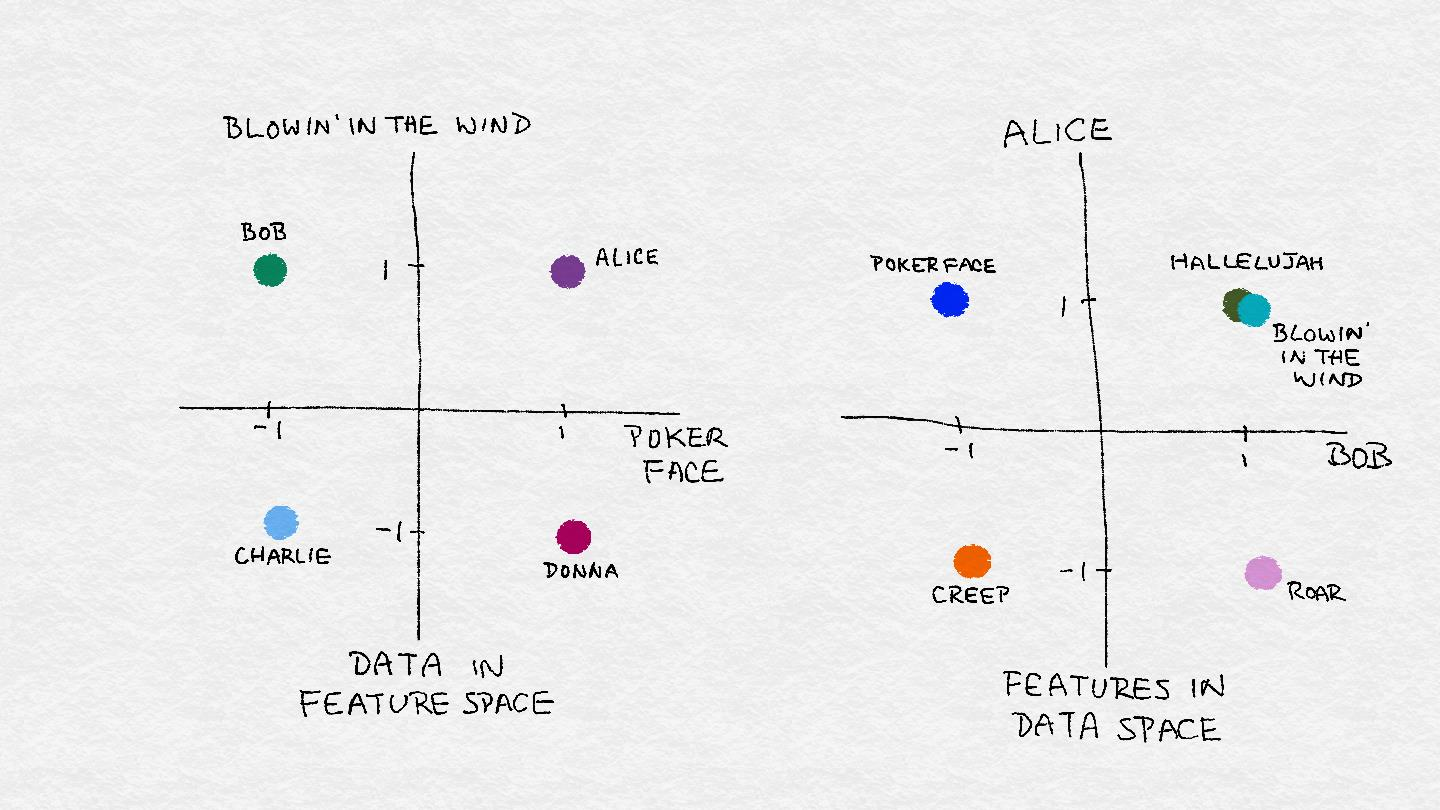Figure 2-2. Illustration of feature space vs. data space.

## 二值化

Million Song 数据集中的用户品味画像包含了一百万个用户在 Echo Nest 的完整音乐聆听历史。下面是有关数据集的一些相关统计数据。

### Echo Nest 品味画像数据集的统计

• 有超过4800万个用户ID、音乐ID和监听计数三元组。
• 完整的数据集包含1019318个独特用户和384546首独特歌曲。
• 引文：Echo Nest 品味画像的数据子集，官方的 Million Song 数据集的用户数据集，可从这里获得：http://labrosa.ee.columbia.edu/millionsong/tasteprofile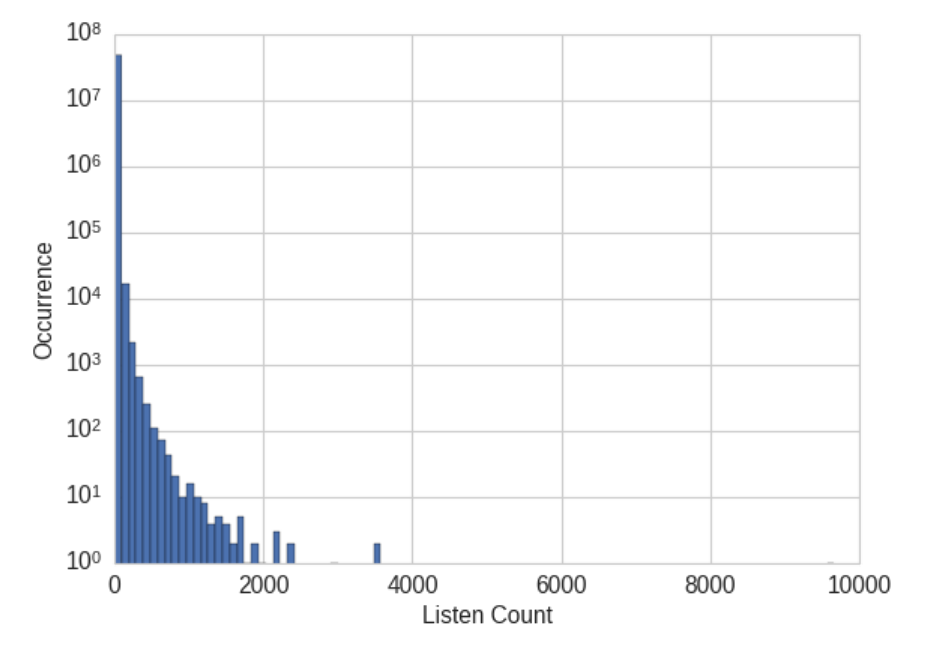Figure 2-3. Histogram of listen counts in the user taste profile of the Million Song Dataset. Note that the y-axis is on a log scale.

### 例子2-1。使 Million Song 数据集中听歌计数二进制化。

import pandas as pd
# The table contains user-song-count triplets. Only non-zero counts are
# included. Hence to binarize the count, we just need to set the entire
# count column to 1.
listen_count = 1


## 量化或装箱

### 关于第 6 轮 Yelp 数据集的统计

• 有782种商户类别。
• 完整的数据集包含 1569264 个（约1.6M）评论和 61184 个（61K）商户。
• “餐厅”（990627个评论）和“夜生活”（210028个评论）是最流行的类别，评论计数是明智的。
• 没有一个商户同时属于餐厅和夜生活分类。因此，这两组评论之间没有重叠。

### 例子2-2。在YELP数据集中可视化商户评论计数。

>>> import pandas as pd
>>> import json
>>> biz_file.close()
>>> import matplotlib.pyplot as plt
>>> import seaborn as sns
### Plot the histogram of the review counts
>>> sns.set_style('whitegrid')
>>> fig, ax = plt.subplots()
>>> biz_df['review_count'].hist(ax=ax, bins=100)
>>> ax.set_yscale('log')
>>> ax.tick_params(labelsize=14)
>>> ax.set_xlabel('Review Count', fontsize=14)
>>> ax.set_ylabel('Occurrence', fontsize=14)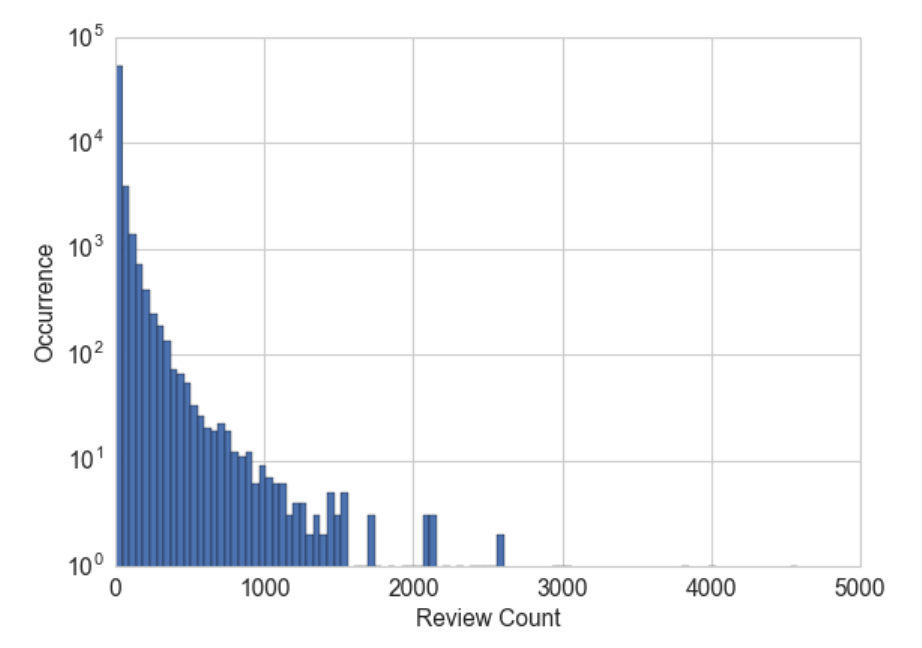Figure 2-4. Histogram of business review counts in the Yelp reviews dataset. The y-axis is on a log-scale.

### 例子2-3。用固定宽度的箱进行量化计数

>>> import numpy as np
### Generate 20 random integers uniformly between 0 and 99
>>> small_counts = np.random.randint(0, 100, 20)
>>> small_counts
array([30, 64, 49, 26, 69, 23, 56, 7, 69, 67, 87, 14, 67, 33, 88, 77, 75, 47, 44, 93])
### Map to evenly spaced bins 0-9 by division
>>> np.floor_divide(small_counts, 10)
array([3, 6, 4, 2, 6, 2, 5, 0, 6, 6, 8, 1, 6, 3, 8, 7, 7, 4, 4, 9], dtype=int32)
### An array of counts that span several magnitudes
>>> large_counts = [296, 8286, 64011, 80, 3, 725, 867, 2215, 7689, 11495, 91897, 44, 28, 7971, 926, 122, 22222]
### Map to exponential-width bins via the log function
>>> np.floor(np.log10(large_counts))
array([ 2., 3., 4., 1., 0., 2., 2., 3., 3., 4., 4., 1., 1., 3., 2., 2., 4.])


### 例子 2-4。计算 Yelp 商户评论数的十分位数

>>> deciles = biz_df['review_count'].quantile([.1, .2, .3, .4, .5, .6, .7, .8, .9])
>>> deciles
0.1 3.0
0.2 4.0
0.3 5.0
0.4 6.0
0.5 8.0
0.6 12.0
0.7 17.0
0.8 28.0
0.9 58.0
Name: review_count, dtype: float64
### Visualize the deciles on the histogram
>>> sns.set_style('whitegrid')
>>> fig, ax = plt.subplots()
>>> biz_df['review_count'].hist(ax=ax, bins=100)
>>> for pos in deciles:
...     handle = plt.axvline(pos, color='r')
>>> ax.legend([handle], ['deciles'], fontsize=14)
>>> ax.set_yscale('log')
>>> ax.set_xscale('log')
>>> ax.tick_params(labelsize=14)
>>> ax.set_xlabel('Review Count', fontsize=14)
>>> ax.set_ylabel('Occurrence', fontsize=14)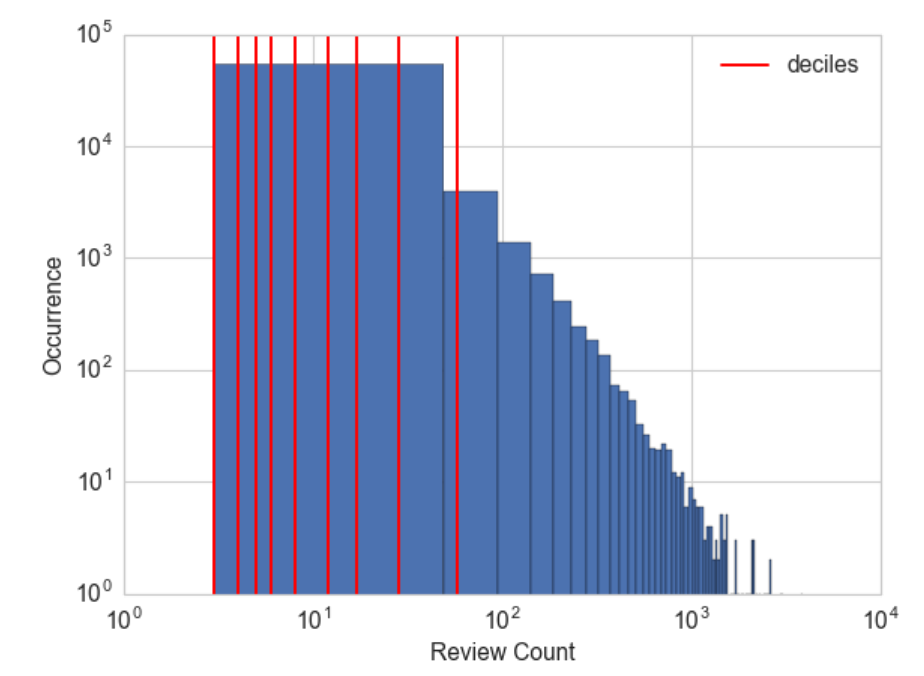Figure 2-5. Deciles of the review counts in the Yelp reviews dataset. Note that both x- and y-axes are in log scale.

### 例子2-5。按分位数分箱计数。

### Continue example Example 2-3 with large_counts
>>> import pandas as pd
### Map the counts to quartiles
>>> pd.qcut(large_counts, 4, labels=False)
array([1, 2, 3, 0, 0, 1, 1, 2, 2, 3, 3, 0, 0, 2, 1, 0, 3], dtype=int64)
### Compute the quantiles themselves
>>> large_counts_series = pd.Series(large_counts)
>>> large_counts_series.quantile([0.25, 0.5, 0.75])
0.25 122.0
0.50 926.0
0.75 8286.0
dtype: float64


## 对数转换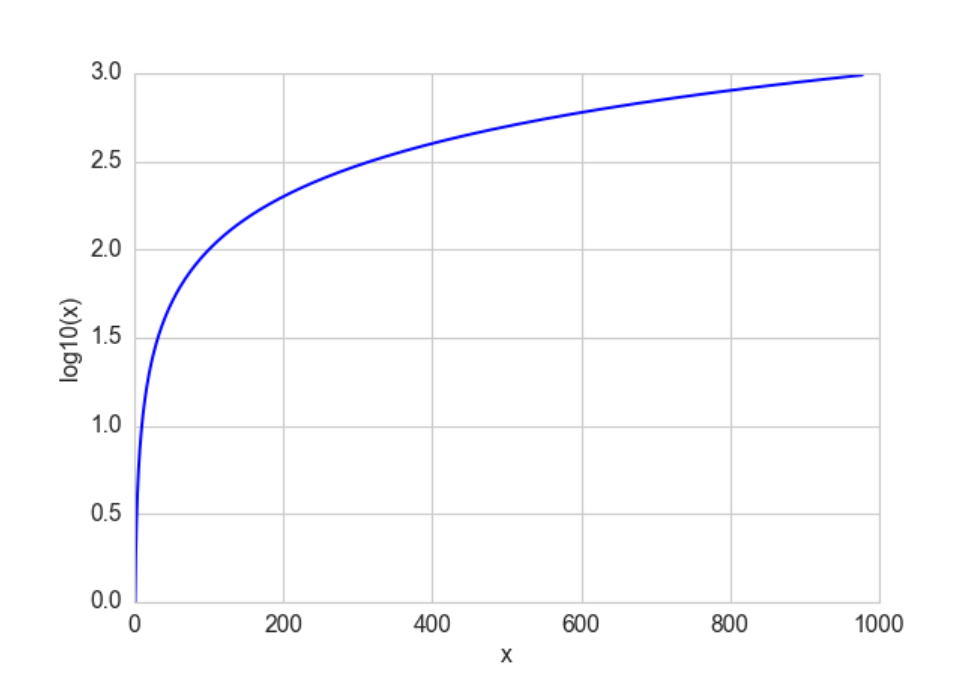Figure 2-6. The log function compresses the high numeric range and expands the low range. Note how the horizontal x values from 100 to 1000 got compressed into just 2.0 to 3.0 in the vertical y range, while the tiny horizontal portion of x values less than 100 are mapped to the rest of the vertical range.

### 例子2-6。可视化对数变换前后评论数分布

fig, (ax1, ax2) = plt.subplots(2,1)
biz_df['review_count'].hist(ax=ax1, bins=100)
ax1.tick_params(labelsize=14)
ax1.set_xlabel('review_count', fontsize=14)
ax1.set_ylabel('Occurrence', fontsize=14)

biz_df['log_review_count'].hist(ax=ax2, bins=100)
ax2.tick_params(labelsize=14)
ax2.set_xlabel('log10(review_count))', fontsize=14)
ax2.set_ylabel('Occurrence', fontsize=14)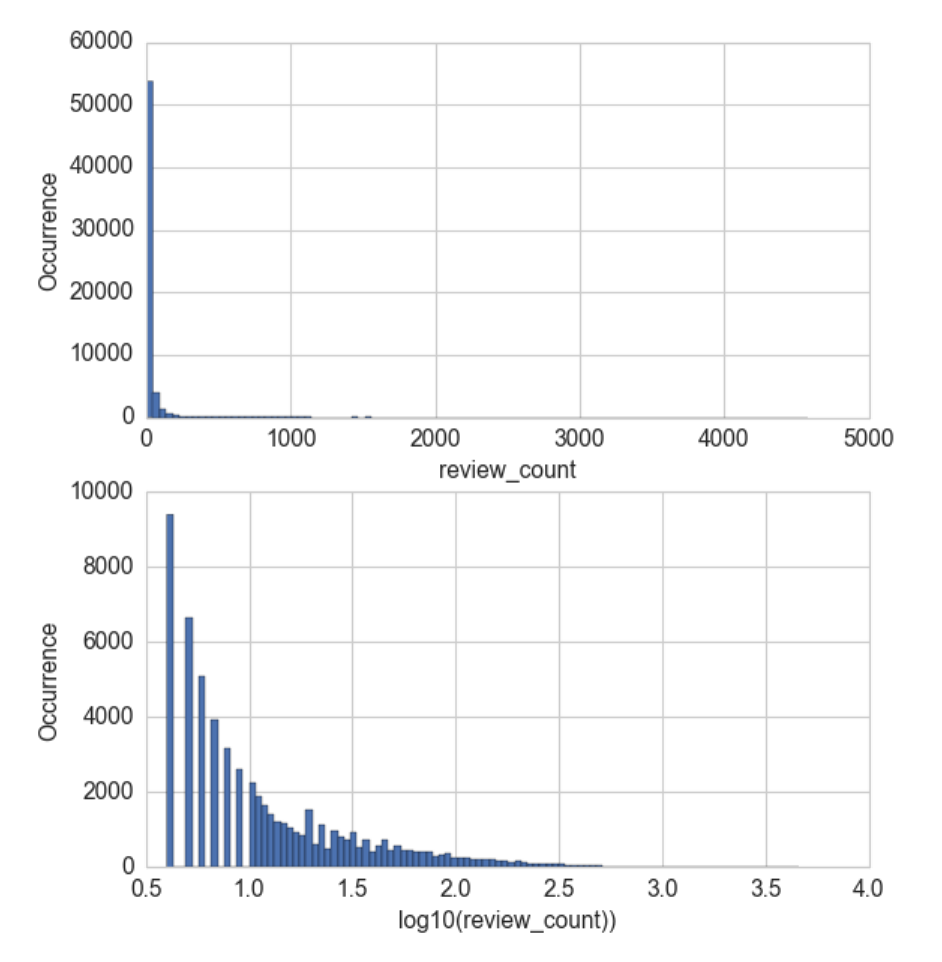Figure 2-7. Comparison of Yelp business review counts before (top) and after (bottom) log transformation.

### 在线新闻流行数据集的统计

• 该数据集包括 MasHabor 在两年的时间内出版的 39797 个新闻文章的60个特征。
• 引证: K. Fernandes, P. Vinagre 和 P. Cortez . 一种用于预测在线新闻的流行程度的主动智能决策支持系统。2015 第十七届 EPIA 活动, 葡萄牙人工智能会议论文集, 9月, 葡萄牙科英布拉。

### 例子2-7。可视化在有对数变换和没有对数变换时新闻文章流行度的分布。

fig, (ax1, ax2) = plt.subplots(2,1)
df['n_tokens_content'].hist(ax=ax1, bins=100)
ax1.tick_params(labelsize=14)
ax1.set_xlabel('Number of Words in Article', fontsize=14)
ax1.set_ylabel('Number of Articles', fontsize=14)

df['log_n_tokens_content'].hist(ax=ax2, bins=100)
ax2.tick_params(labelsize=14)
ax2.set_xlabel('Log of Number of Words', fontsize=14)
ax2.set_ylabel('Number of Articles', fontsize=14)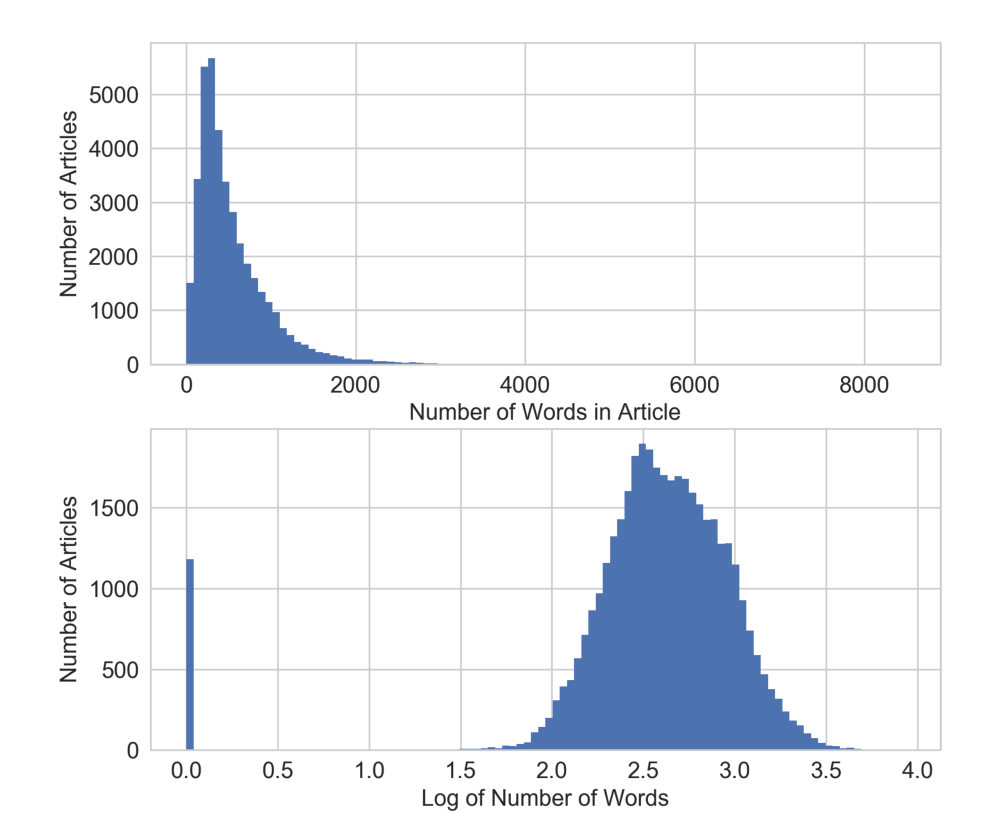Figure 2-8. Comparison of word counts in Mashable news articles before (top) and after (bottom) log transformation.

## 对数转换实战

### 例子2-8。使用对数转换 YELP 评论数预测平均商户评级

>>> import pandas as pd
>>> import numpy as np
>>> import json
>>> from sklearn import linear_model
>>> from sklearn.model_selection import cross_val_score

## Using the previously loaded Yelp reviews dataframe,
## compute the log transform of the Yelp review count.
## Note that we add 1 to the raw count to prevent the logarithm from
## exploding into negative infinity in case the count is zero.
>>> biz_df['log_review_count'] = np.log10(biz_df['review_count'] + 1)

## Train linear regression models to predict the average stars rating of a business,
## using the review_count feature with and without log transformation
## Compare the 10-fold cross validation score of the two models
>>> m_orig = linear_model.LinearRegression()
>>> scores_orig = cross_val_score(m_orig, biz_df[['review_count']], biz_df['stars'], cv=10)
>>> m_log = linear_model.LinearRegression()
>>> scores_log = cross_val_score(m_log, biz_df[['log_review_count']], biz_df['stars'], cv=10)
>>> print("R-squared score without log transform: %0.5f (+/- %0.5f)" % (scores_orig.mean(), scores_orig.std() * 2))
>>> print("R-squared score with log transform: %0.5f (+/- %0.5f)" % (scores_log.mean(), scores_log.std() * 2))
R-squared score without log transform: -0.03683 (+/- 0.07280)
R-squared score with log transform: -0.03694 (+/- 0.07650)


### 例子2-9。利用经过对数转换在线新闻数据中的词数量预测文章流行度

## Download the Online News Popularirty dataset from UCI, then use
## Pandas to load the file into a dataframe
>>> df = pd.read_csv('OnlineNewsPopularity.csv', delimiter=', ')

## Take the log transform of the 'n_tokens_content' feature, which
## represents the number of words (tokens) in a news article.
>>> df['log_n_tokens_content'] = np.log10(df['n_tokens_content'] + 1)

## Train two linear regression models to predict the number of shares
## of an article, one using the original feature and the other the
## log transformed version.
>>> m_orig = linear_model.LinearRegression()
>>> scores_orig = cross_val_score(m_orig, df[['n_tokens_content']], df['shares'], cv=10)
>>> m_log = linear_model.LinearRegression()
>>> scores_log = cross_val_score(m_log, df[['log_n_tokens_content']], df['shares'], cv=10)
>>> print("R-squared score without log transform: %0.5f (+/- %0.5f)" % (scores_orig.mean(), scores_orig.std() * 2))
>>> print("R-squared score with log transform: %0.5f (+/- %0.5f)" % (scores_log.mean(), scores_log.std() * 2))
R-squared score without log transform: -0.00242 (+/- 0.00509)
R-squared score with log transform: -0.00114 (+/- 0.00418)


### 示例2-10。可视化新闻流程度预测问题中输入输出相关性。

fig2, (ax1, ax2) = plt.subplots(2,1)
ax1.scatter(df['n_tokens_content'], df['shares'])
ax1.tick_params(labelsize=14)
ax1.set_xlabel('Number of Words in Article', fontsize=14)
ax1.set_ylabel('Number of Shares', fontsize=14)

ax2.scatter(df['log_n_tokens_content'], df['shares'])
ax2.tick_params(labelsize=14)
ax2.set_xlabel('Log of the Number of Words in Article', fontsize=14)
ax2.set_ylabel('Number of Shares', fontsize=14)Figure 2-9. Scatter plot of number of words (input) vs. number of shares (target) in the Online News dataset. The top plot visualizes the original feature, and the bottom plot shows the scatter plot after log transformation.

### 例2-11。可视化 Yelp 商户评论预测中输入与输出的相关性。

fig, (ax1, ax2) = plt.subplots(2,1)
ax1.scatter(biz_df['review_count'], biz_df['stars'])
ax1.tick_params(labelsize=14)
ax1.set_xlabel('Review Count', fontsize=14)
ax1.set_ylabel('Average Star Rating', fontsize=14)

ax2.scatter(biz_df['log_review_count'], biz_df['stars'])
ax2.tick_params(labelsize=14)
ax2.set_xlabel('Log of Review Count', fontsize=14)
ax2.set_ylabel('Average Star Rating', fontsize=14)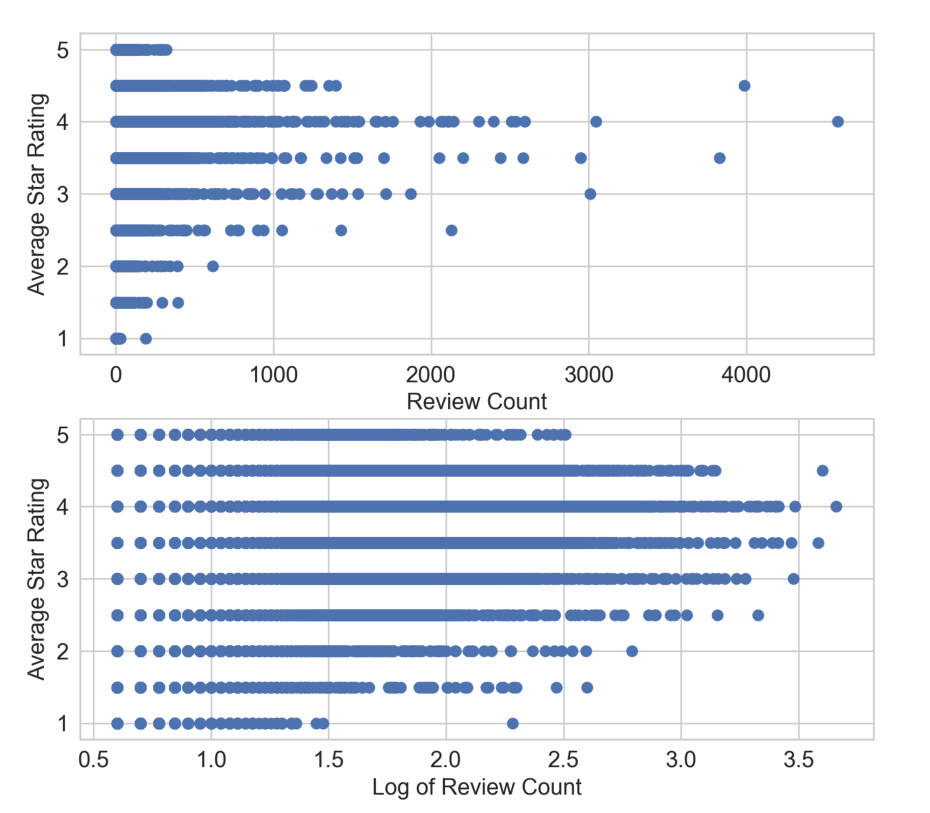Figure 2-10. Scatter plot of review counts (input) vs. average star ratings (target) in the Yelp Reviews dataset. The top panel plots the original review count, and the bottom panel plots the review count after log transformation.

## 数据可视化的重要性

### 功率变换：对数变换的推广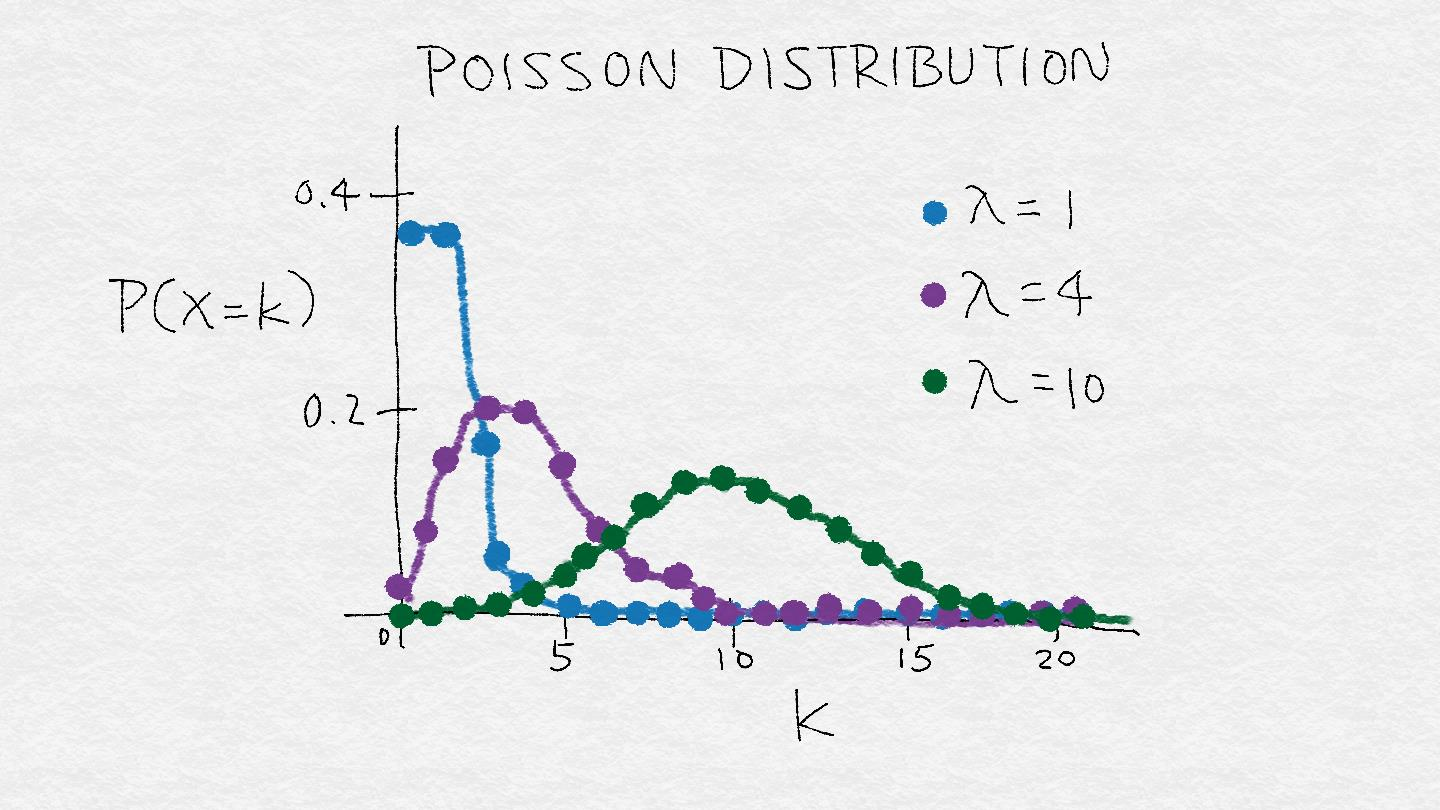Figure 2-11. A rough illustration of the Poisson distribution. λ represents the mean of the distribution. As λ increases, not only does the mode of of the distribution shift to the right, but the mass spreads out and the variance becomes larger. The Poisson distribution is an example distribution where the variance increases along with the mean.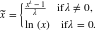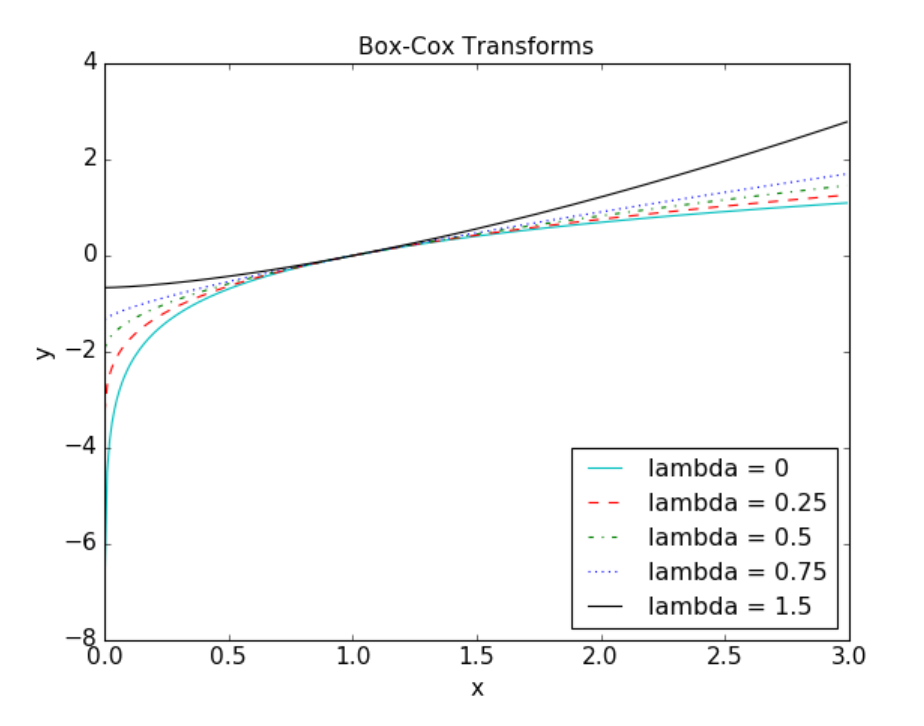Figure 2-12. Box-Cox transforms for different values of λ.

### 例子2-12。Yelp商户评论数的 Box-Cox 变换。

>>> from scipy import stats

# Continuing from the previous example, assume biz_df contains
# the Yelp business reviews data
# Box-Cox transform assumes that input data is positive.
# Check the min to make sure.
>>> biz_df['review_count'].min()
3

# Setting input parameter lmbda to 0 gives us the log transform (without constant offset)
>>> rc_log = stats.boxcox(biz_df['review_count'], lmbda=0)
# By default, the scipy implementation of Box-Cox transform finds the lmbda parameter
# that will make the output the closest to a normal distribution
>>> rc_bc, bc_params = stats.boxcox(biz_df['review_count'])
>>> bc_params
-0.4106510862321085


### 例2-13。可视化评论数的原始、对数转换和 Box-Cox 转换的直方图。

fig, (ax1, ax2, ax3) = plt.subplots(3,1)
# original review count histogram
biz_df['review_count'].hist(ax=ax1, bins=100)
ax1.set_yscale('log')
ax1.tick_params(labelsize=14)
ax1.set_title('Review Counts Histogram', fontsize=14)
ax1.set_xlabel('')
ax1.set_ylabel('Occurrence', fontsize=14)

# review count after log transform
biz_df['rc_log'].hist(ax=ax2, bins=100)
ax2.set_yscale('log')
ax2.tick_params(labelsize=14)
ax2.set_title('Log Transformed Counts Histogram', fontsize=14)
ax2.set_xlabel('')
ax2.set_ylabel('Occurrence', fontsize=14)

# review count after optimal Box-Cox transform
biz_df['rc_bc'].hist(ax=ax3, bins=100)
ax3.set_yscale('log')
ax3.tick_params(labelsize=14)
ax3.set_title('Box-Cox Transformed Counts Histogram', fontsize=14)
ax3.set_xlabel('')
ax3.set_ylabel('Occurrence', fontsize=14)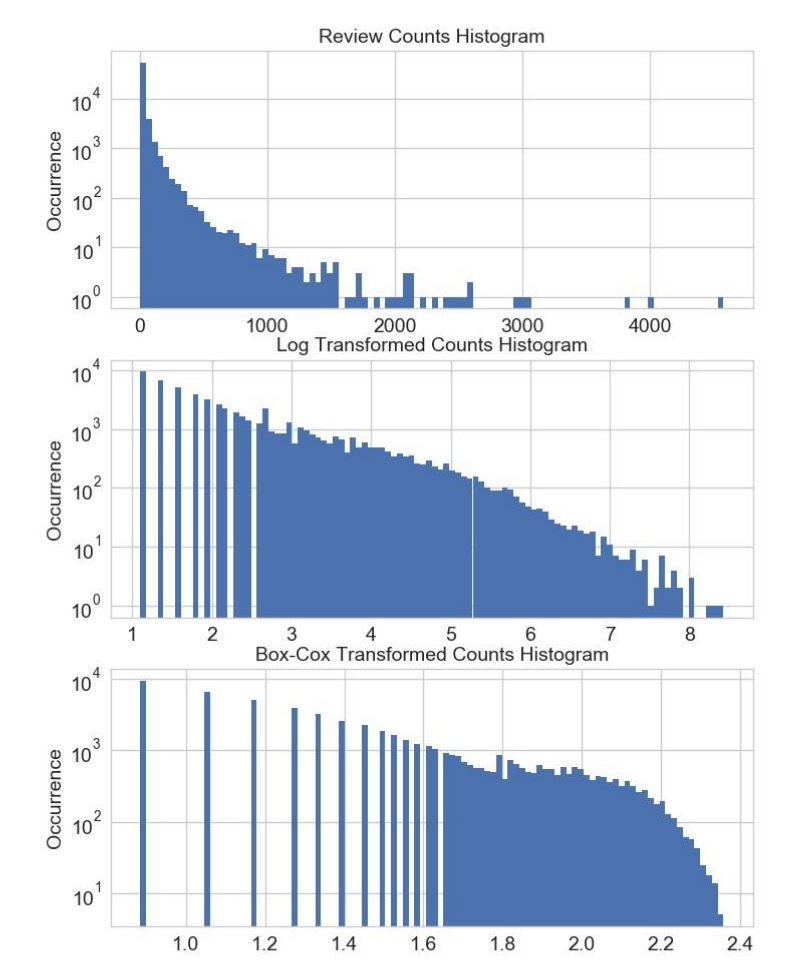Figure 2-13. Box-Cox transformation of Yelp business review counts.

### 例子 2-14。原始和变换后的数据相对正态分布的概率图。

fig2, (ax1, ax2, ax3) = plt.subplots(3,1)
prob1 = stats.probplot(biz_df['review_count'], dist=stats.norm, plot=ax1)
ax1.set_xlabel('')
ax1.set_title('Probplot against normal distribution')
prob2 = stats.probplot(biz_df['rc_log'], dist=stats.norm, plot=ax2)
ax2.set_xlabel('')
ax2.set_title('Probplot after log transform')
prob3 = stats.probplot(biz_df['rc_bc'], dist=stats.norm, plot=ax3)
ax3.set_xlabel('Theoretical quantiles')
ax3.set_title('Probplot after Box-Cox transform')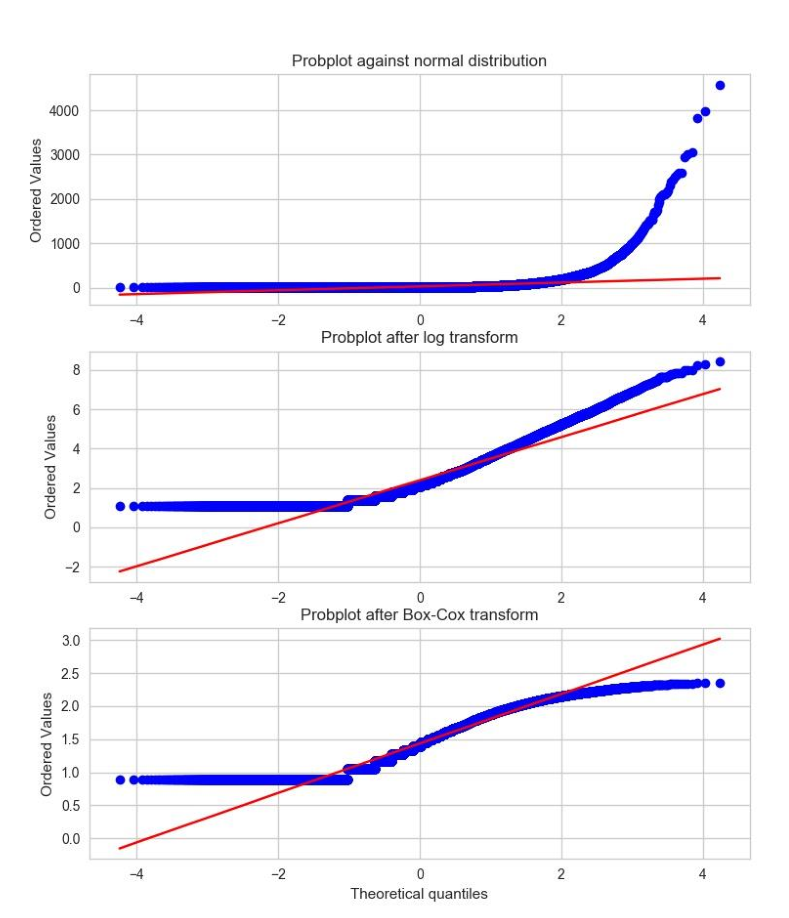Figure 2-14. Comparing the distribution of raw and transformed review counts against the Normal distribution.

## 特征缩放或归一化

### Min-max缩放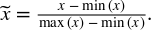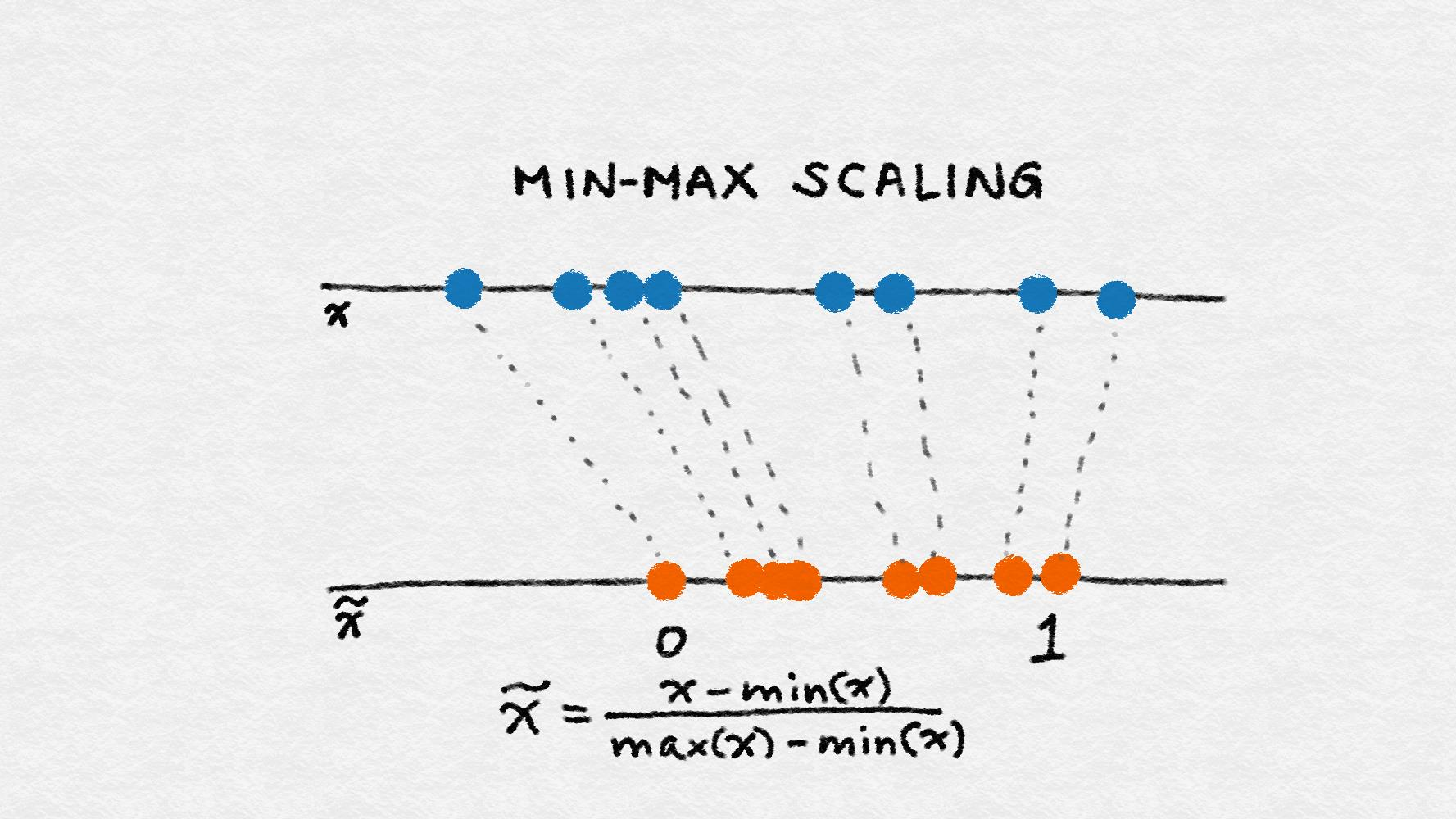Figure 2-15. Min-max scaling

### 标准化（方差缩放）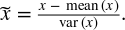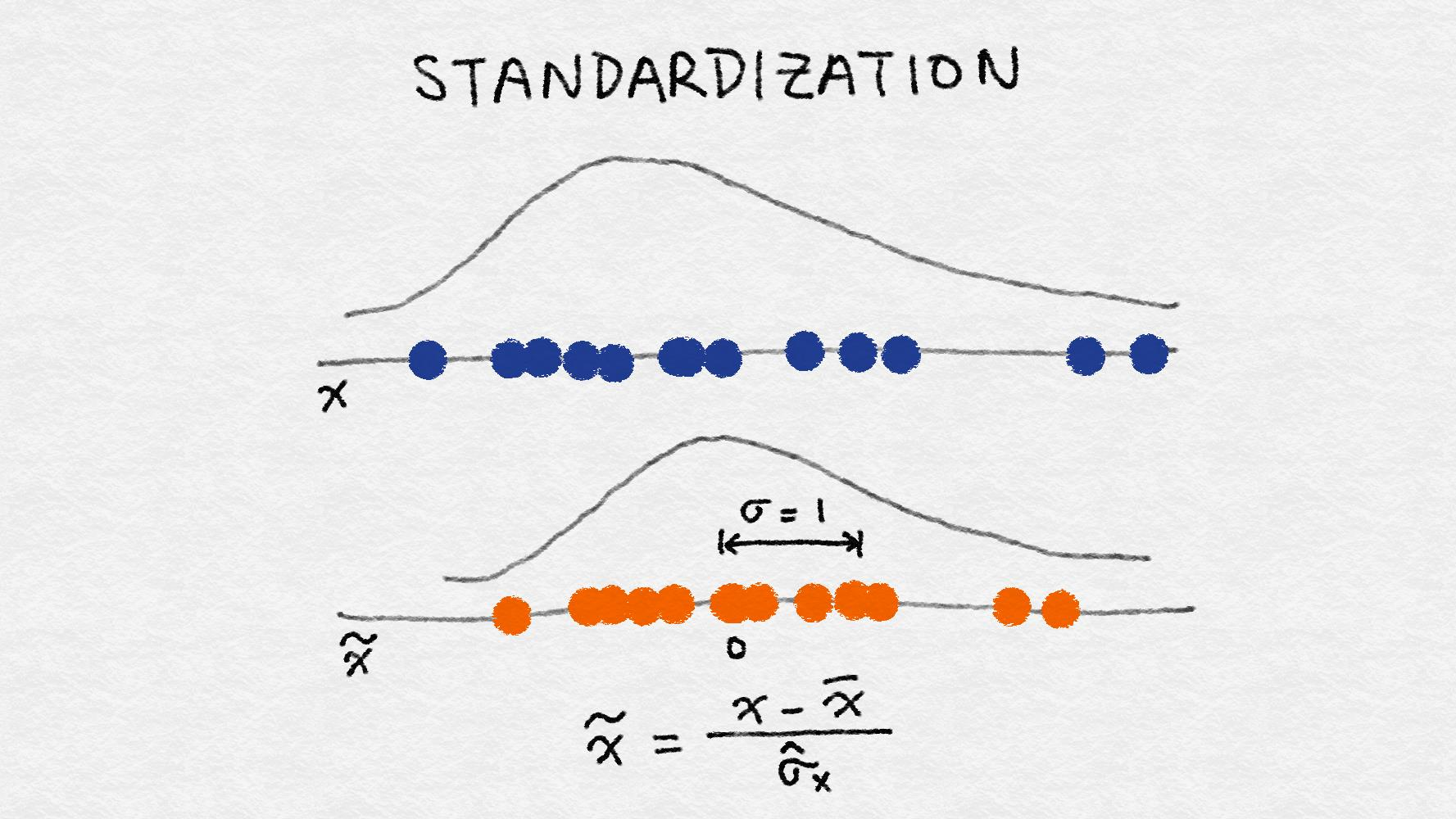Figure 2-16. Illustration of feature standardization

## 不要中心化稀疏数据

### L2 normalization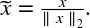L2范数度量向量在坐标空间中的长度。这个定义可以从众所周知的勾股定理中得到，给出三角形两边的长度，可以得到斜边长度。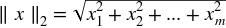L2 范数将求特征的各数据点的平方和, 然后取平方根。L2 规范化后, 该特征列具有范数1。它也可以称为 L2 缩放。(不严谨的说, 缩放意味着和常量相乘, 而规范化可能涉及许多操作。）图2-17说明了 L2 规范化。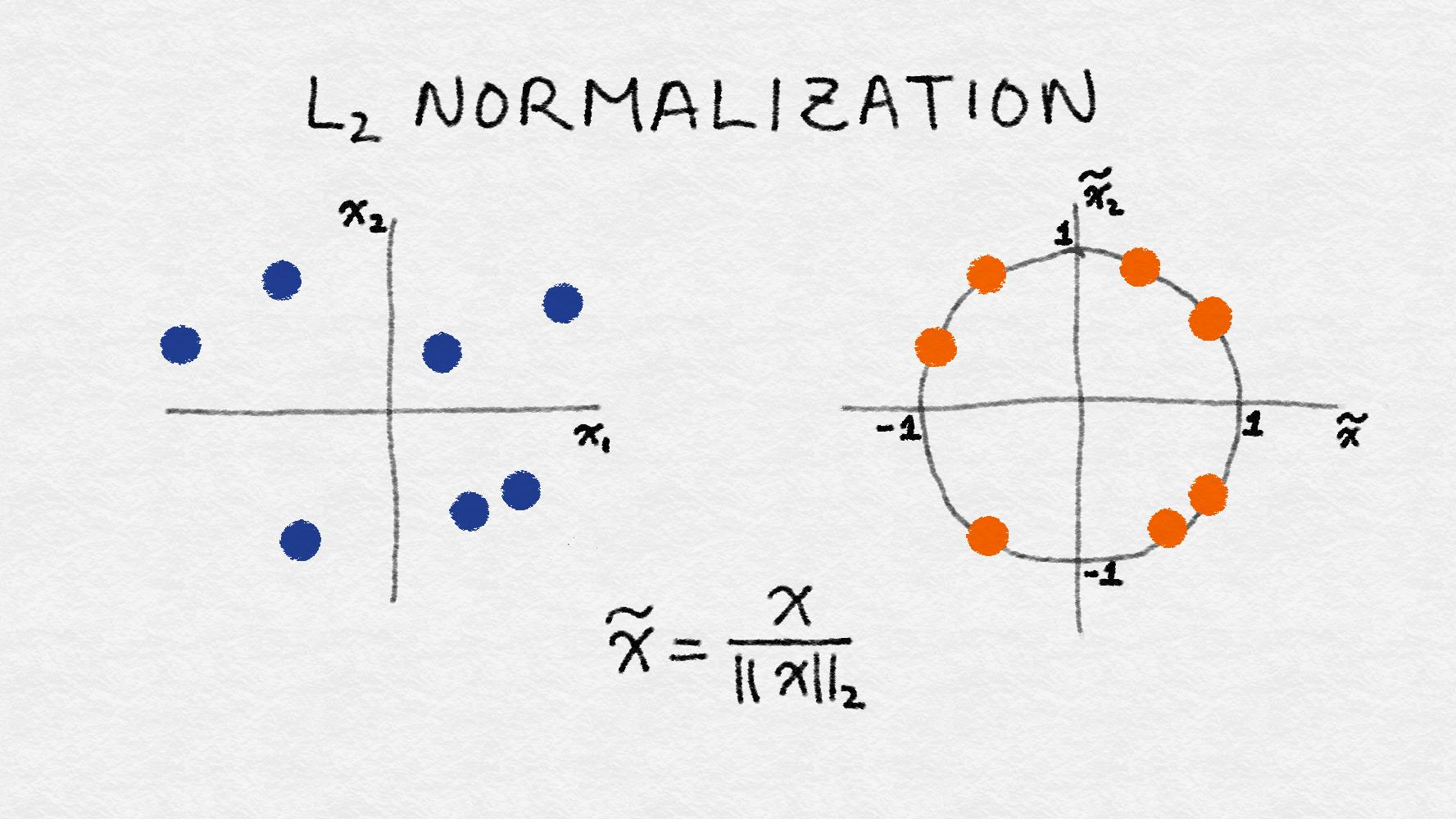Figure 2-17. Illustration of L2 feature normalization

## 数据空间与特征空间

### 例子 2-15。特征缩放示例。

>>> import pandas as pd
>>> import sklearn.preprocessing as preproc

# Load the online news popularity dataset
>>> df = pd.read_csv('OnlineNewsPopularity.csv', delimiter=', ')

# Look at the original data - the number of words in an article
>>> df['n_tokens_content'].as_matrix()
array([ 219., 255., 211., ..., 442., 682., 157.])

# Min-max scaling
>>> df['minmax'] = preproc.minmax_scale(df[['n_tokens_content']])
>>> df['minmax'].as_matrix()
array([ 0.02584376, 0.03009205, 0.02489969, ..., 0.05215955,
0.08048147, 0.01852726])

# Standardization - note that by definition, some outputs will be negative
>>> df['standardized'] = preproc.StandardScaler().fit_transform(df[['n_tokens_content']])
>>> df['standardized'].as_matrix()
array([-0.69521045, -0.61879381, -0.71219192, ..., -0.2218518 ,
0.28759248, -0.82681689])

# L2-normalization
>>> df['l2_normalized'] = preproc.normalize(df[['n_tokens_content']], axis=0)
>>> df['l2_normalized'].as_matrix()
array([ 0.00152439, 0.00177498, 0.00146871, ..., 0.00307663,
0.0047472 , 0.00109283])


### 例2-16。绘制原始数据和缩放数据的直方图。

>>> fig, (ax1, ax2, ax3, ax4) = plt.subplots(4,1)
>>> fig.tight_layout()

>>> df['n_tokens_content'].hist(ax=ax1, bins=100)
>>> ax1.tick_params(labelsize=14)
>>> ax1.set_xlabel('Article word count', fontsize=14)
>>> ax1.set_ylabel('Number of articles', fontsize=14)

>>> df['minmax'].hist(ax=ax2, bins=100)
>>> ax2.tick_params(labelsize=14)
>>> ax2.set_xlabel('Min-max scaled word count', fontsize=14)
>>> ax2.set_ylabel('Number of articles', fontsize=14)

>>> df['standardized'].hist(ax=ax3, bins=100)
>>> ax3.tick_params(labelsize=14)
>>> ax3.set_xlabel('Standardized word count', fontsize=14)
>>> ax3.set_ylabel('Number of articles', fontsize=14)

>>> df['l2_normalized'].hist(ax=ax4, bins=100)
>>> ax4.tick_params(labelsize=14)
>>> ax4.set_xlabel('L2-normalized word count', fontsize=14)
>>> ax4.set_ylabel('Number of articles', fontsize=14)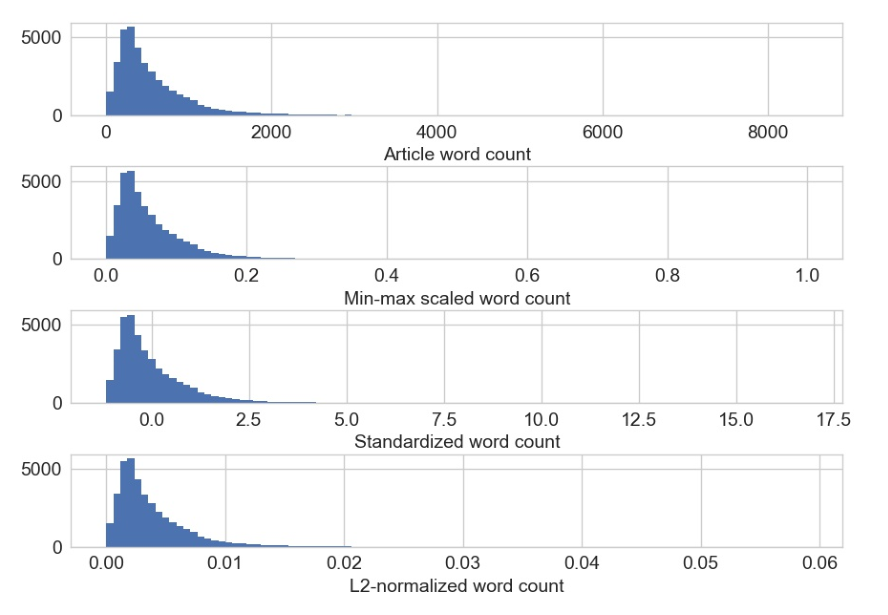Figure 2-18. Original and scaled news article word counts. Note that only the scale of the x-axis changes; the shape of the distribution stays the same with feature scaling.

## 交互特征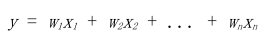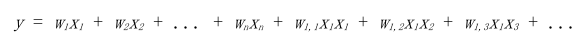### 例子 2--17。用于预测的交互特征示例。

>>> from sklearn import linear_model
>>> from sklearn.model_selection import train_test_split
>>> import sklearn.preprocessing as preproc

### Assume df is a Pandas dataframe containing the UCI online news dataset
>>> df.columns
Index(['url', 'timedelta', 'n_tokens_title', 'n_tokens_content',
'n_unique_tokens', 'n_non_stop_words', 'n_non_stop_unique_tokens',
'num_hrefs', 'num_self_hrefs', 'num_imgs', 'num_videos',
'average_token_length', 'num_keywords', 'data_channel_is_lifestyle',
'data_channel_is_entertainment', 'data_channel_is_bus',
'data_channel_is_socmed', 'data_channel_is_tech',
'data_channel_is_world', 'kw_min_min', 'kw_max_min', 'kw_avg_min',
'kw_min_max', 'kw_max_max', 'kw_avg_max', 'kw_min_avg', 'kw_max_avg',
'kw_avg_avg', 'self_reference_min_shares', 'self_reference_max_shares',
'self_reference_avg_sharess', 'weekday_is_monday', 'weekday_is_tuesday',
'weekday_is_wednesday', 'weekday_is_thursday', 'weekday_is_friday',
'weekday_is_saturday', 'weekday_is_sunday', 'is_weekend', 'LDA_00',
'LDA_01', 'LDA_02', 'LDA_03', 'LDA_04', 'global_subjectivity',
'global_sentiment_polarity', 'global_rate_positive_words',
'global_rate_negative_words', 'rate_positive_words',
'rate_negative_words', 'avg_positive_polarity', 'min_positive_polarity',
'max_positive_polarity', 'avg_negative_polarity',
'min_negative_polarity', 'max_negative_polarity', 'title_subjectivity',
'title_sentiment_polarity', 'abs_title_subjectivity',
'abs_title_sentiment_polarity', 'shares'],
dtype='object')

### Select the content-based features as singleton features in the model,
### skipping over the derived features
features = ['n_tokens_title', 'n_tokens_content',
'n_unique_tokens', 'n_non_stop_words', 'n_non_stop_unique_tokens',
'num_hrefs', 'num_self_hrefs', 'num_imgs', 'num_videos',
'average_token_length', 'num_keywords', 'data_channel_is_lifestyle',
'data_channel_is_entertainment', 'data_channel_is_bus',
'data_channel_is_socmed', 'data_channel_is_tech',
'data_channel_is_world']

>>> X = df[features]
>>> y = df[['shares']]

### Create pairwise interaction features, skipping the constant bias term
>>> X2 = preproc.PolynomialFeatures(include_bias=False).fit_transform(X)
>>> X2.shape
(39644, 170)

### Create train/test sets for both feature sets
>>> X1_train, X1_test, X2_train, X2_test, y_train, y_test = train_test_split(X, X2, y, test_size=0.3, random_state=123)

>>> def evaluate_feature(X_train, X_test, y_train, y_test):
...     '''Fit a linear regression model on the training set and score on the test set'''
...     model = linear_model.LinearRegression().fit(X_train, y_train)
...     r_score = model.score(X_test, y_test)
...     return (model, r_score)

### Train models and compare score on the two feature sets
>>> (m1, r1) = evaluate_feature(X1_train, X1_test, y_train, y_test)
>>> (m2, r2) = evaluate_feature(X2_train, X2_test, y_train, y_test)
>>> print("R-squared score with singleton features: %0.5f" % r1)
>>> print("R-squared score with pairwise features: %0.10f" % r2)
R-squared score with singleton features: 0.00924
R-squared score with pairwise features: 0.0113276523


## 特征选择

Filtering（过滤）: 预处理可以删除那些不太可能对模型有用的特征。例如，可以计算每个特征与响应变量之间的相关或相互信息，并筛除相关信息或相互信息低于阈值的特征。第3章讨论了文本特征的过滤技术的例子。过滤比下面的包装（wrapper）技术便宜得多，但是他们没有考虑到正在使用的模型。因此他们可能无法为模型选择正确的特征。最好先保守地进行预过滤，以免在进行模型训练步骤之前无意中消除有用的特征。

Wrapper methods（包装方法）：这些技术是昂贵的，但它们允许您尝试特征子集，这意味着你不会意外删除自身无法提供信息但在组合使用时非常有用的特征。包装方法将模型视为提供特征子集质量分数的黑盒子。shi一个独立的方法迭代地改进子集。

Embedded methods（嵌入式方法）：嵌入式方法执行特征选择作为模型训练过程的一部分。 例如，决策树固有地执行特征选择，因为它在每个训练步骤选择一个要在其上进行树分裂的特征。另一个例子是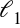正则，它可以添加到任何线性模型的训练目标中。鼓励模型使用一些特征而不是许多特征。因此它也被称为模型的稀疏约束。嵌入式方法将特征选择作为模型训练过程的一部分。它们不如包装方法那么强大，但也远不如包装方法那么昂贵。与过滤相比，嵌入式方法会选择特定于模型的特征。从这个意义上讲，嵌入式方法在计算费用和结果质量之间取得平衡。

## 参考书目

Guyon, Isabell, and André Elisseeff. 2003. Journal of Machine Learning Research Special Issue on Variable and Feature Selection. 3(Mar):1157--1182.

Johnston, Jack, and John DiNardo. 1997. Econometric Methods (Fourth Edition). New York: McGraw Hill.